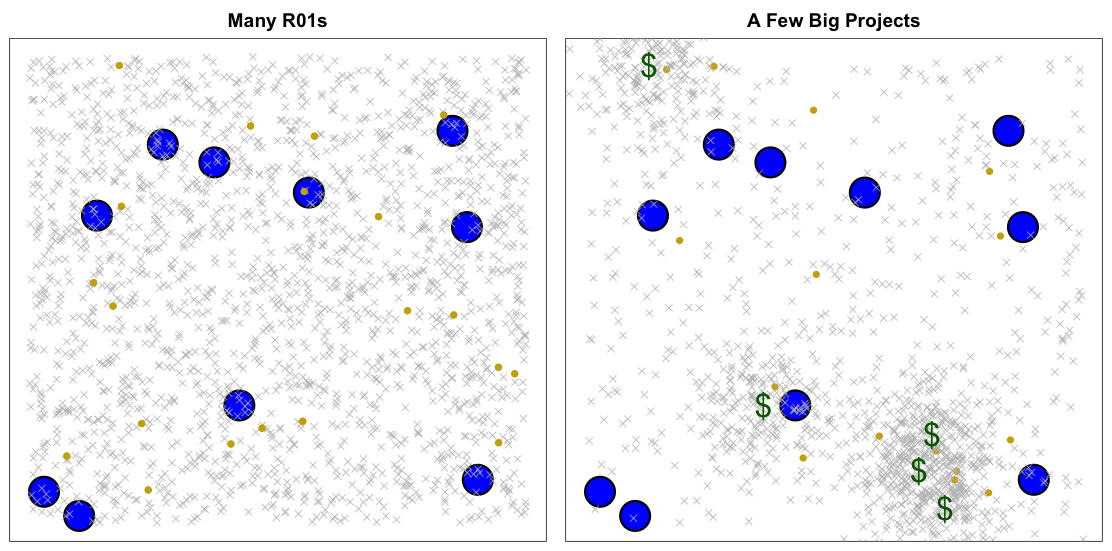# Thinking like a statistician: the importance of investigator-initiated grants

A substantial amount of scientific research is funded by investigator-initiated grants. A researcher has an idea, writes it up and sends a proposal to a funding agency. The agency then elicits help from a group of peers to evaluate competing proposals. Grants are awarded to the most highly ranked ideas. The percent awarded depends on how much funding gets allocated to these types of proposals. At the NIH, the largest funding agency of these types of grants, the success rate recently fell below 20% from a high above 35%. Part of the reason these percentages have fallen is to make room for large collaborative projects. Large projects seem to be increasing, and not just at the NIH. In Europe, for example, the Human Brain Project has an estimated cost of over 1 billion US$over 10 years. To put this in perspective, 1 billion dollars can fund over 500 NIH R01s. R01 is the NIH mechanism most appropriate for investigator initiated proposals. The merits of big science has been widely debated (for example here and here). And most agree that some big projects have been successful. However, in this post I present a statistical argument highlighting the importance of investigator-initiated awards. The idea is summarized in the graph below.The two panes above represent two different funding strategies: fund-many-R01s (left) or reduce R01s to fund several large projects (right). The grey crosses represent investigators and the gold dots represent potential paradigm-shifting geniuses. Location on the Cartesian plane represent research areas, with the blue circles denoting areas that are prime for an important scientific advance. The largest scientific contributions occur when a gold dot falls in a blue circle. Large contributions also result from the accumulation of incremental work produced by grey crosses in the blue circles. Although not perfect, the peer review approach implemented by most funding agencies appears to work quite well at weeding out unproductive researchers and unpromising ideas. They also seem to do well at spreading funds across general areas. For example NIH spreads funds across diseases and public health challenges (for example cancer, mental health, heart, genomics, heart and lung disease.) as well as general medicine, genomics and information. However, precisely predicting who will be a gold dot or what specific area will be a blue circle seems like an impossible endeavor. Increasing the number of tested ideas and researchers therefore increases our chance of success. When a funding agency decides to invest big in a specific area (green dollar signs) they are predicting the location of a blue circle. As funding flows into these areas, so do investigators (note the clusters). The total number of funded lead investigators also drops. The risk here is that if the dollar sign lands far from a blue dot, we pull researchers away from potentially fruitful areas. If after 10 years of funding, the Human Brain Project doesn’t “achieve a multi-level, integrated understanding of brain structure and function” we will have missed out on trying out 500 ideas by hundreds of different investigators. With a sample size this large, we expect at least a handful of these attempts to result in the type of impactful advance that justifies funding scientific research. The simulation presented (code below) here is clearly an over simplification, but it does depict the statistical reason why I favor investigator-initiated grants. The simulation clearly depicts that the strategy of funding many investigator-initiated grants is key for the continued success of scientific research. set.seed(2) library(rafalib) thecol="gold3" mypar(1,2,mar=c(0.5,0.5,2,0.5)) ## Start with the many R01s model ### ##generate location of 2,000 investigators N = 2000 x = runif(N) y = runif(N) ## 1% are geniuses Ng = N*0.01 g = rep(4,N);g[1:Ng]=16 ## generate location of important areas of research M0 = 10 x0 = runif(M0) y0 = runif(M0) r0 = rep(0.03,M0) ##Make the plot nullplot(xaxt="n",yaxt="n",main="Many R01s") symbols(x0,y0,circles=r0,fg="black",bg="blue",lwd=3,add=TRUE,inches=FALSE) points(x,y,pch=g,col=ifelse(g==4,"grey",thecol)) points(x,y,pch=g,col=ifelse(g==4,NA,thecol)) ### Generate the location of 5 big projects M1 = 5 x1 = runif(M1) y1 = runif(M1) ##make initial plot nullplot(xaxt="n",yaxt="n",main="A Few Big Projects") symbols(x0,y0,circles=r0,fg="black",bg="blue",lwd=3,add=TRUE,inches=FALSE) ### Generate location of investigators attracted ### to location of big projects. There are 1000 total ### investigators Sigma = diag(2)*0.005 N1 = 200 Ng1 = round(N1*0.01) g1 = rep(4,N);g1[1:Ng1]=16 library(MASS) for(i in 1:M1){ xy = mvrnorm(N1,c(x1[i],y1[i]),Sigma) points(xy[,1],xy[,2],pch=g1,col=ifelse(g1==4,"grey",thecol)) } ### generate location of investigators that ignore big projects ### note now 500 instead of 200. Note overall total ## is also less because large projects result in less ## lead investigators N = 500 x = runif(N) y = runif(N) Ng = N*0.01 g = rep(4,N);g[1:Ng]=16 points(x,y,pch=g,col=ifelse(g==4,"grey",thecol)) points(x1,y1,pch="$",col="darkgreen",cex=2,lwd=2)